Enquiry Cart: 0 product(s)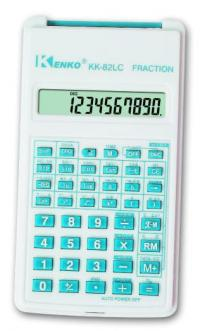Scientific Calculator, 56 functions (KK-82LC)
KK-82LC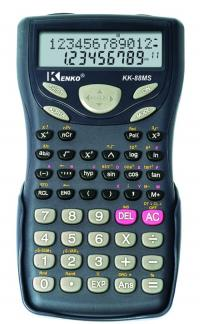Scientific Calculator, 240 functions (KK-88MS)
KK-88MSScientific Calculator, 240 functions (KK-80MS)
KK-80MS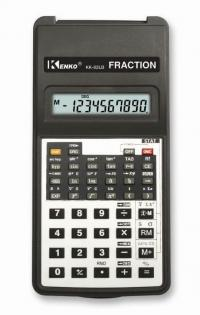Scientific Calculator, 56 functions (KK-82LBN)
KK-82LBN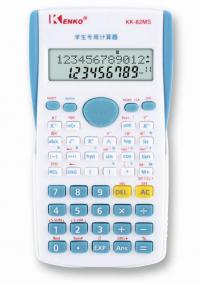Scientific Calculator, 240 functions (KK-82MS)
KK-82MSScientific Calculator, 240 functions (KK-82MS-A)
KK-82MS-AScientific Calculator, 240 functions (KK-82MS-B)
KK-82MS-B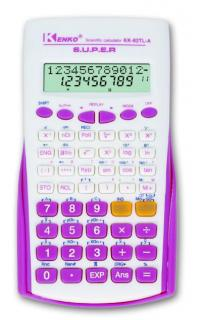Scientific Calculator, 229 functions (KK-82TL-A)
KK-82TL-AScientific Calculator, 56 functions (KK-104)
KK-104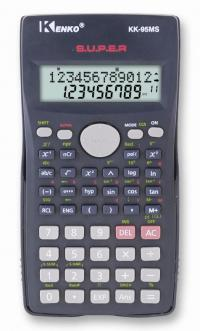Scientific Calculator, 240 functions (KK-95MS)
KK-95MS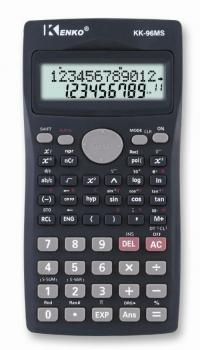Scientific Calculator, 240 functions (KK-96MS)
KK-96MSScientific Calculator, 56 functions (KK-105B)
KK-105B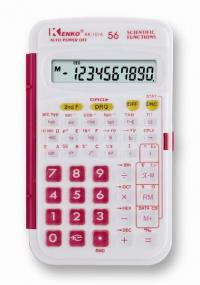Scientific Calculator, 56 functions (KK-107A)
KK-107A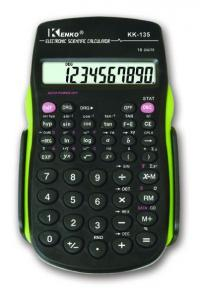Scientific Calculator, 56 functions (KK-135)
KK-135Scientific Calculator, 56 functions (KK-220)
KK-220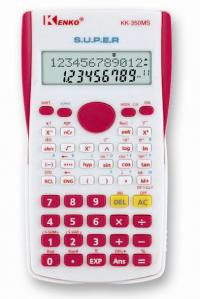Scientific Calculator, 240 functions (KK-350MS)
KK-350MSScientific Calculator, 56 functions (KK-1006)
KK-1006Scientific Calculator, 240 functions (KK-3950MS)
KK-3950MS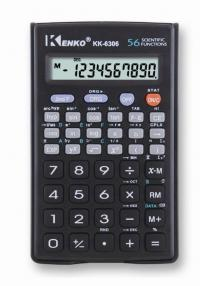Scientific Calculator, 56 functions (KK-6306)
KK-6306Scientific Calculator, 56 functions (KK-9300)
KK-9300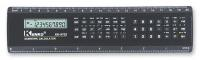Scientific Rule Calculator, 56 functions (KK-9702)
KK-9702Scientific Calculator, 56 functions (KK-F92)
KK-F92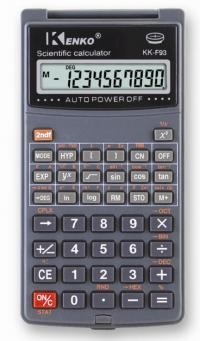Scientific Calculator, 56 functions (KK-F93)
KK-F93Scientific Calculator, 240 functions (KK-98MS)
KK-98MS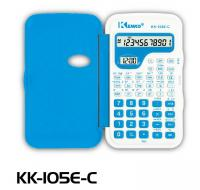Scientific Calculator, 56 functions (KK-105E)
KK-105EScientific Calculator, 240 functions (KK-82MS-G)
KK-82MS-G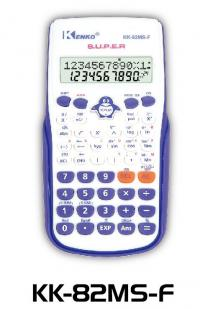Scientific Calculator, 240 functions (KK-82MS-F)
KK-82MS-F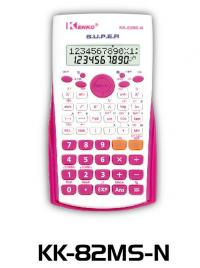Scientific Calculator, 240 functions (KK-82MS-N)
KK-82MS-N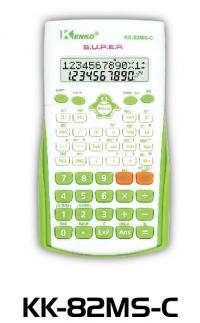Scientific Calculator, 240 functions (KK-82MS-C)
KK-82MS-C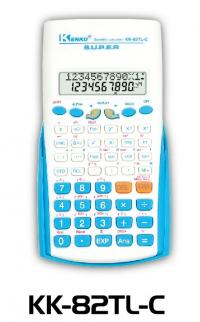Scientific Calculator, 229 functions (KK-82TL-C)
KK-82TL-CScientific Calculator, 229 functions (KK-82TL-N)
KK-82TL-N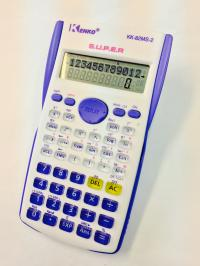Scientific Calculator, 240 functions (KK-82MS-2
KK-82MS-2Scientific Calculator, 240 functions (KK-991ES)
KK-991ES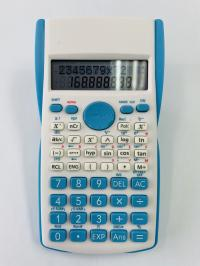Scientific Calculator, 240 functions (KK-9308)
KK-9308Scientific Calculator, 229 functions (KK-911W)
KK-911W